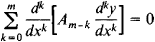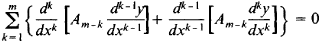a differential equation that has the same solutions as its adjoint equation. An ordinary self-adjoint differential equation of even order 2m has the formand of odd order 2m—1 has the formwhere the Ai are functions of x. The concept of self-adjoint differential equations plays an important role in the theory of ordinary and partial differential equations. Under certain boundary conditions, the lefthand part of a self-adjoint differential equation defines a self-adjoint differential operator. Second-order self-adjoint differential equations are the most important in applications.

Mentioned in ?
References in periodicals archive ?
However, dynamic modal characteristics for nonuniform beams can be found in [28, 29] in which self-adjoint differential equations and the method of factorization [30-32] have been used.
Caruntu, "Self-adjoint differential equations for classical orthogonal polynomials," Journal of Computational and Applied Mathematics, vol.
 Walter Leighton, On self-adjoint differential equations of second order, J.

Site: Follow: Share:
Open / Close